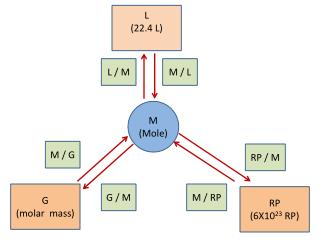DownloadDownload PresentationG (molar mass)

# G (molar mass)

Télécharger la présentation## G (molar mass)

- - - - - - - - - - - - - - - - - - - - - - - - - - - E N D - - - - - - - - - - - - - - - - - - - - - - - - - - -
##### Presentation Transcript

1. L (22.4 L) L / M M / L M (Mole) M / G RP / M G (molar mass) G / M M / RP RP (6X1023 RP)

2. Things you must know: 1 mole =the molar mass of a substance (G) 1 mole =6.02 X 1023 representative particles (RP) (atoms, molecules, FU) 1 mole =22.4 liters of a gas (L)

3. Other things you must know • Polyatomic ions • Group B ions (cuprous, ferric, etc.) • Writing formulas from names • Balancing ionic formula charges • Scientific notation • Representative particles (atoms, molecules, FU) • Read chapter 6 and 7

4. How to start a problem • Write the balanced formulas • Calculate the molar mass • Determine the given – put it on the left • Determine the unit of what you are looking for – put it on the right • Fill in the center with conversion factors

5. How many grams in 200 L of NO2?

6. How many liters in 1.8 X 1027 molecules of NO2?

7. Determine the volume in liters of 2.8 moles of NO2.

8. Find the number of moles in 150 grams of cuprous oxide.

9. How many atoms are in 5.12 moles of magnesium phosphate? (scientific notation)

10. How many liters in 238 g CO2?

11. How many liters in 238 g CO2? 238 G 1 1 M 44 G 22.4 L 1 M 121 L

12. How many grams in 1.806 X1025 formula units of aluminum oxide ? 1.806 X1025 FU 1 1 M 6.02X1023 FU 102 G 1 M 3060 G

13. How many molecules in .0684 l nitrogen gas?

14. How many molecules in .0684 l nitrogen gas? .0684 L 1 1 M 22.4 L 6X1023 FU 1 M 1.8 X 1021 FU